# Cartesian & Polar Coordinate Systems

In this article, we describe the two types of coordinate systems used in a plane: the Cartesian and the polar coordinate systems. These coordinate systems are utilized by this graphing calculator and other graphers on this site.

Assuming you are familiar with one-dimensional coordinate systems, such as a number line or axis, we will focus on two-dimensional coordinate systems. A two-dimensional coordinate system, also known as a coordinate plane, is used to locate an ordered pair (a, b) in a plane.

The Cartesian coordinate system is formed by two intersecting axes with a common origin. The axis containing the values of the first coordinate is usually drawn horizontally, while the other is drawn vertically. These axes are commonly denoted by the letters x and y and are called the x-axis and y-axis, respectively.

On the other hand, the polar coordinate system uses one reference axis, usually horizontal, called the polar axis. Its origin is called the pole. Instead of a second axis, the polar coordinate system uses angles measured from the polar axis about the pole.

The following figures illustrate the Cartesian coordinate system and the polar coordinate system used in a plane.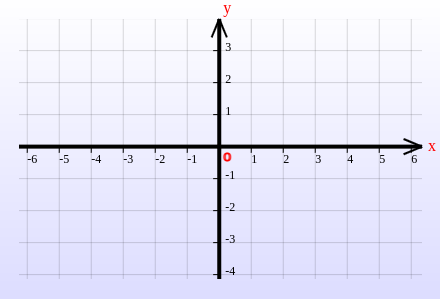Fig 1. Cartesian coordinate system The Cartesian coordinate system uses a horizontal axis and a vertical axis to locate points in a plane.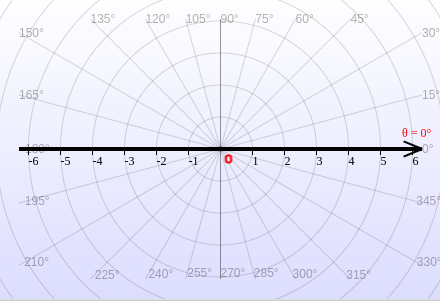Fig 2. Polar coordinate system The polar coordinate system uses a distance from the origin and an angle from a reference direction to locate points in a plane.

Both the Cartesian coordinate system and polar coordinate system can be used to locate or plot points. By plotting points one can graph functions (what is the graph of a function?), graph equations and graph parametric curves. The following figures illustrate how the point (a, b) is plotted in each coordinate system for a = 3 and b = 2.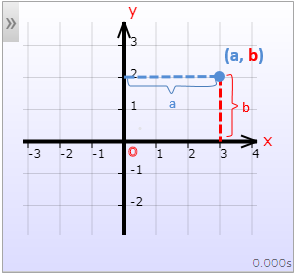Fig 3. Cartesian plot: a = 3, b = 2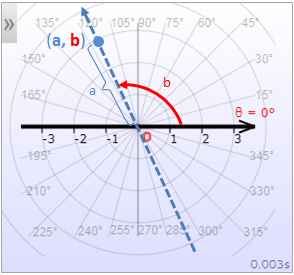Fig 4. Polar plot: a = 3, b = 2 (≃ 115°)

In the Cartesian coordinate system with the x-axis and y-axis as previously described, the first coordinate, a, is referred to as the x-coordinate (or abscissa) while the second coordinate, b, is called the y-coordinate (or ordinate).

In the polar coordinate system, a is referred to as the radial coordinate, while b is known as the angular coordinate or polar angle. The radial coordinate represents the signed distance from the pole, while the angular coordinate represents the angle by which the polar axis must be rotated counterclockwise to locate the point (a, b).

In the Cartesian coordinate system, the point (a, b) can be described as x = a and y = b. In the polar coordinate system, it is customary to use r and θ (or φ) to represent the point, where r = a and θ = b (or φ = b).

Every ordered pair (a, b) has a unique location in a coordinate plane. However, a location (point) in the polar coordinate system can have multiple polar representations. This is because adding multiples of 2π (or 360°) to an angle does not change its "location". Therefore, the ordered pairs (a, b+2π) and (a, b) determine the same point in the polar coordinate plane. Similarly, adding π to the angular coordinate and changing the radial coordinate from a to -a also determines the same point as (a, b), as shown in the following illustrations:

In summary, all the ordered pairs (a, b), (a, b+2nπ) and (-a, b+(2n+1)π) identify the same point in the polar coordinate system for integers n.

If the axes are perpendicular, as shown above, the Cartesian coordinate system in a plane is called the rectangular coordinate system or orthogonal coordinate system. However, the axes need not be perpendicular, in which case, we have a non-perpendicular coordinate system, as demonstrated in the following figures. Note that the polar axis in the polar coordinate system need not be horizontal.

## Conversion between Polar and Cartesian Coordinates

An ordered pair (a, b) plotted in the Cartesian coordinate system generally has a different location from when plotted in the polar coordinate system (with the same origin and pole). Given the coordinates of a point in one coordinate system, you might ask what its coordinates are in the other coordinate system so that both identify the same point. To answer this question, we assume that in the Cartesian coordinate system, the independent axis is horizontal and the dependent axis is vertical. We also assume that the polar axis coincides with the x-axis, shares the same origin, and both Cartesian axes and the polar axis have the same scales.

### Converting Polar Coordinates to Cartesian Coordinates

Converting polar coordinates to Cartesian coordinates is straightforward using the definitions of trigonometric functions on a trig circle. Referring to the following figure, it is clear that the point (r, φ) in the polar coordinate system and the point (rcos(φ), rsin(φ)) in the Cartesian coordinate system coincide.Fig 10. Polar to Cartesian: x = rcos(φ), y = rsin(φ)

So we have the following formula for converting polar coordinates to Cartesian coordinates:

Polar to Cartesian Coordinates

x = r*cos(φ) y = r*sin(φ)

Note: The above conversion formula holds even if r is negative. If r < 0, then x = -|r|*cosφ = -(-r)*cosφ = r*cosφ, and similarly, y = -|r|*sinφ = -(-r)*sinφ = r*sinφ.

### Converting Cartesian Coordinates to Polar Coordinates

As shown in the following figure, regardless of the location of the point (x, y), we have r =√(x2 + y2) and tan(φ) = y/x by the Pythagorean Theorem.

Because tan-1() always gives an "angle" between -π/2 and π/2, we have φ = tan-1(y/x) if (x, y) is in the first or fourth quadrant (that is, if x > 0) by the definition of tan-1.

Because we are dividing by x, x must be non-zero. For the case where x = 0, the point is on the y-axis and the angular coordinate is θ = π/2 and the radial coordinate is r = y.

If (x, y) is in the second or third quadrant (that is, if x < 0), we must add (or subtract) π to tan-1(y/x) to obtain the corresponding polar angle.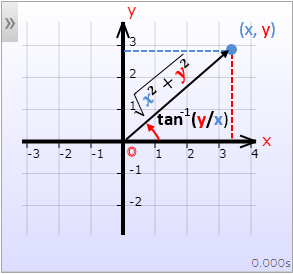Fig 11. Cartesian to Polar: r = √(x2+y2), φ = tan-1(y/x)

To summarize:

Cartesian to Polar Coordinates (1)

If x = 0
r = y φ = π/2
Otherwise
r = √(x2+y2)
φ = {
tan-1(y/x)      if x > 0 tan-1(y/x)+π if x < 0

Alternatively, when x < 0, instead of adding π/2 to tan-1(y/x), we can multiply r by -1 to get the corresponding radial coordinate.

Cartesian to Polar Coordinates (2)

If x = 0
r = y φ = π/2
Otherwise
r = {
√(x2+y2) if x > 0 -√(x2+y2) if x < 0
φ= tan-1(y/x)

The radial coordinate in the above can be expressed concisely using the signum function, sgn(), as:

Cartesian to Polar Coordinates

If x = 0
r = y φ = π/2
Otherwise
r = sgn(x)√(x2+y2) φ = tan-1(y/x)

NOTE: Each of the above three formulas can be used to convert Cartesian to polar coordinates.